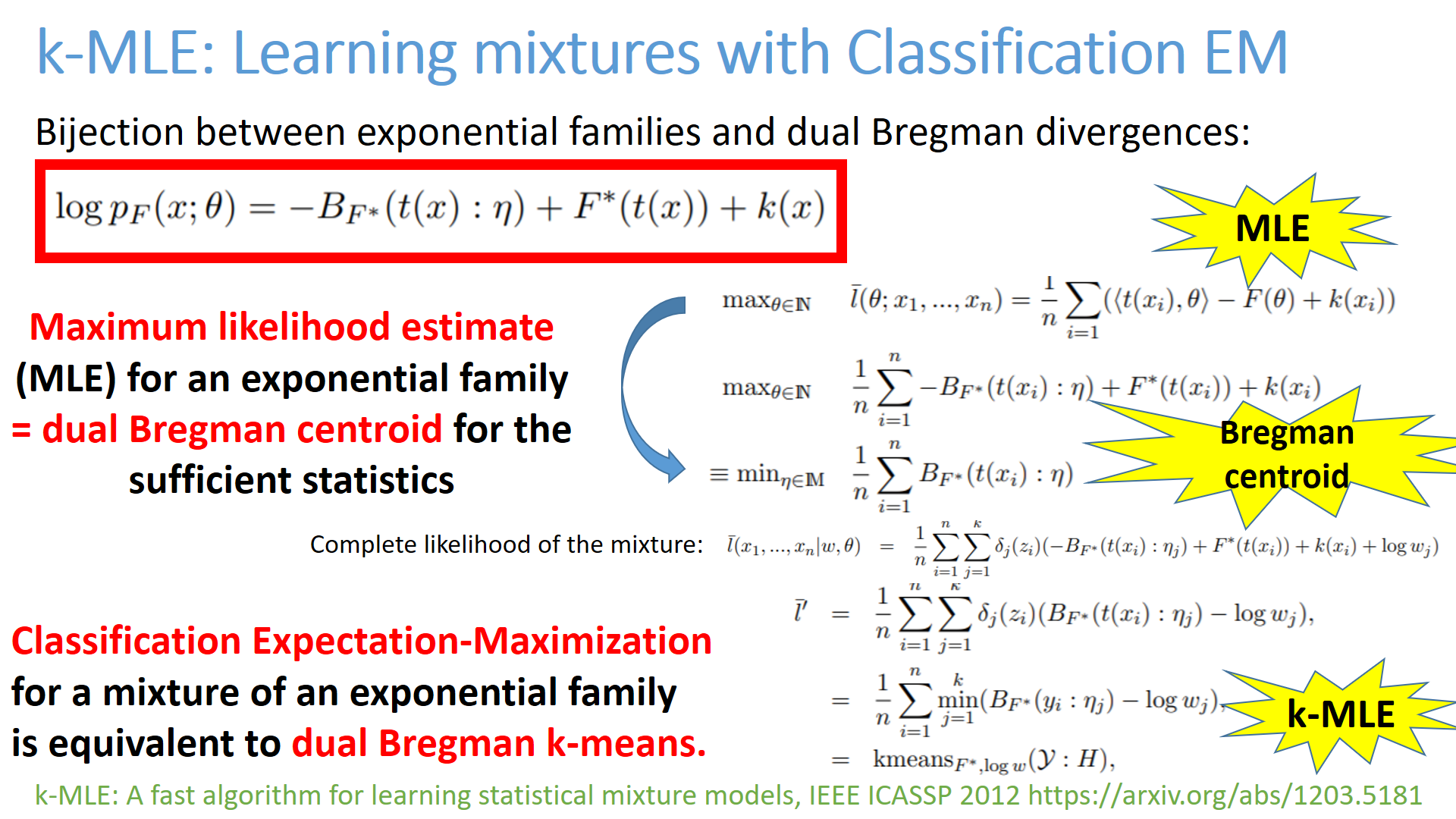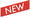# Information geometry and divergences

## Foundations, Applications, and Software APIs

Historically, Information Geometry (IG, tutorials, textbooks and monographs) aimed at unravelling the geometric structures of families of probability distributions called the statistical models.
A statistical model can either be

• parametric (eg., family of normal distributions),
• semi-parametric (eg., family of Gaussian mixture models) or
• non-parametric (family of mutually absolutely continuous smooth densities).
A parametric statistical model is said regular when the Fisher information matrix is positive-definite (and well-defined). Otherwise, the statistical model is irregular (eg., infinite Fisher information and semi-positive definite Fisher information when the model is not identifiable).

The Fisher-Rao manifold of a statistical parametric model is a Riemannian manifold equipped with the Fisher information metric. The geodesic length on a Fisher-Rao manifold is called Rao's distance [Hotelling 1930][Rao 1945]. More generally, Amari proposed the dualistic structure of IG which consists of a pair of torsion-free affine connections coupled to the Fisher metric [Amari 1980's]. Given a dualistic structure, we can build generically a one-parameter family of dualistic information-geometric structures, called the α-geometry. When both connections are flat, the information-geometric space is said dually flat: For example, the Amari's ±1-structures of exponential families and mixture families are famous examples of dually flat spaces in information geometry. In differential geometry, geodesics are defined as autoparallel curves with respect to a connection. When using the default Levi-Civita metric connection derived from the Fisher metric on Fisher-Rao manifolds, we get Rao's distance which are locally minimizing geodesics. Eguchi showed how to build from any smooth distortion (originally called a contrast function) a dualistic structure: The information geometry of divergences [Eguchi 1982]. The information geometry of Bregman divergences yields dually flat spaces: It is a special cases of Hessian manifolds which are differentiable manifolds equipped with a metric tensor being a Hessian metric and a flat connection [Shima 2007]. Since geometric structures scaffold spaces independently of any applications, these pure information-geometric Fisher-Rao structure and α-structures of statistical models can also be used in non-statistical contexts too: For example, for analyzing interior point methods with barrier functions in optimization, or for studying time-series models, etc.

Statistical divergences between parametric statistical models amount to parameter divergences on which we can use the Eguchi's divergence information geometry to get a dualistic structure. A projective divergence is a divergence which is invariant by independent rescaling of its parameters. A statistical projective divergence is thus useful for estimating computationally intractable statistical models (eg., gamma divergences, Cauchy-Schwarz divergence and Hölder divergences, or singly-sided projective Hyvärinen divergence). A conformal divergence is a divergence scaled by a conformal factor which may depend on one or two of its arguments. The metric tensor obtained from Eguchi's information divergence of a conformal divergence is a conformal metric of the metric obtained from the divergence, hence its name. By analogy to total least squares vs least squares, a total divergence is a divergence which is invariant wrt. to rotations (eg., total Bregman divergences). An important property of divergences on the probability simplex is to be monotone by coarse-graining. That is, merging bins and considering reduced histograms should give a distance less or equal than the distance on the full resolution histograms. This information monotonicity property holds for f-divergences (called invariant divergences in information geometry), Hilbert log cross-ratio distance, or Aitchison distance for example. Some statistical divergences are upper bounded (eg., Jensen-Shannon divergence) while others are not (eg., Jeffreys' divergence). Optimal transport distances require a ground base distance on the sample space. A diversity index generalizes a two-point distance to a family of parameters/distributions. It usually measures the dispersion around a center point (eg., like variance measures the dispersion around the centroid).

## Finsler manifolds

Finsler manifolds are proposed to model irregular parametric statistical models (where Fisher information can be infinite)

## Exponential families and Mixture families

Continuous or discrete exponential families
• Online k-MLE for Mixture Modeling with Exponential Families, GSI 2015
• k-MLE: A fast algorithm for learning statistical mixture models, IEEE ICASSP 2012• Fast Learning of Gamma Mixture Models with k-MLE, SIMBAD 2013: 235-249
• k-MLE for mixtures of generalized Gaussians, ICPR 2012: 2825-2828
• Simplification and hierarchical representations of mixtures of exponential families, Signal Process. 90(12): 3197-3212 (2010)
•The analytic dually flat space of the statistical mixture family of two prescribed distinct Cauchy components
• On the Geometry of Mixtures of Prescribed Distributions, IEEE ICASSP 2018

## Information geometry of deformed exponential families

q-deformed exponential families, q-Gaussians, etc.

## Information geometry of the probability simplex

• Clustering in Hilbert simplex geometry [project page]
• Geometry of the probability simplex and its connection to the maximum entropy method, Journal of Applied Mathematics, Statistics and Informatics 16(1):25-35, 2020
Bruhat-Tits space
open access (publisher)

## Information geometry of singular statistical models

•A Geometric Modeling of Occam's Razor in Deep Learning
•Towards Modeling and Resolving Singular Parameter Spaces using Stratifolds, Neurips OPT workshop 2021

## Hilbert geometry

Hilbert geometry are induced by a bounded convex open domain. Hilbert geometry generalize the Klein model of hyperbolic geometry and the Cayley-Klein geometry Beware that Hilbert geometry are never Hilbert spaces!